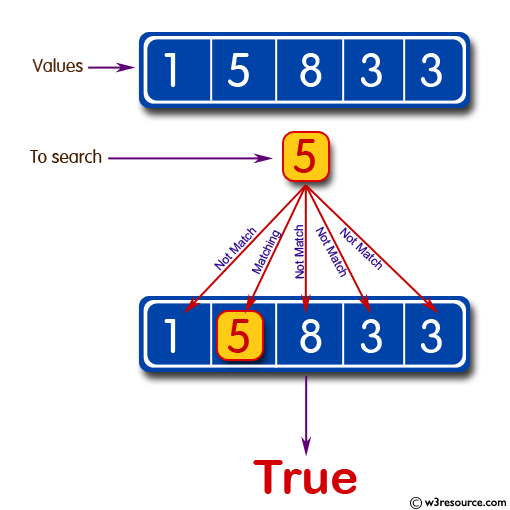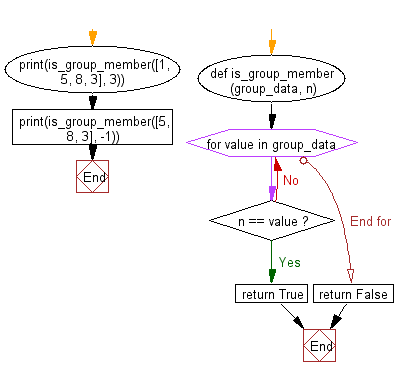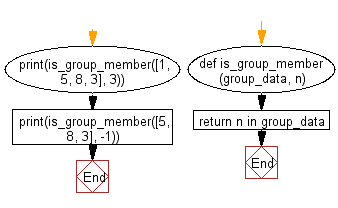﻿ Python: Check whether a specified value is contained in a group of values - w3resource# Python: Check whether a specified value is contained in a group of values

## Python Basic: Exercise-25 with Solution

Write a Python program to check whether a specified value is contained in a group of values.

Test Data:
3 -> [1, 5, 8, 3] : True
-1 -> [1, 5, 8, 3] : False

Pictorial Presentation:Sample Solution-1:

Python Code:

``````def is_group_member(group_data, n):
for value in group_data:
if n == value:
return True
return False
print(is_group_member([1, 5, 8, 3], 3))
print(is_group_member([5, 8, 3], -1))
```
```

Sample Output:

```True
False
```

Flowchart:## Visualize Python code execution:

The following tool visualize what the computer is doing step-by-step as it executes the said program:

Sample Solution-2:

Python Code:

``````def is_group_member(group_data, n):
return n in group_data
print(is_group_member([1, 5, 8, 3], 3))
print(is_group_member([5, 8, 3], -1))
```
```

Sample Output:

```True
False
```

Flowchart:## Visualize Python code execution:

The following tool visualize what the computer is doing step-by-step as it executes the said program:

Python Code Editor:

Have another way to solve this solution? Contribute your code (and comments) through Disqus.

What is the difficulty level of this exercise?

Test your Python skills with w3resource's quiz

﻿

## Python: Tips of the Day

List comprehension:

```>>> m = [x ** 2 for x in range(5)]
>>> m
[0, 1, 4, 9, 16]
```